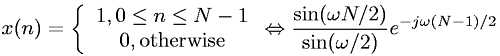Equations > Signal Processing > Fourier Transform Pairs > Discrete-Time Fourier transform of a boxcar sequence

### Discrete-Time Fourier transform of a boxcar sequenceLatex Code:

MathML Code:

 $x\left(n\right)=\left\{\begin{array}{c}\hfill 1,0\le n\le N-1\hfill \\ \hfill 0,\mathrm{otherwise}\hfill \end{array}⇔\frac\mathrm{sin}\left(\omega N/2\right)\mathrm{sin}\left(\omega /2\right){e}^{-j\omega \left(N-1\right)/2}$

MathType 5.0: